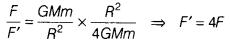# Calculate the average density of the Earth

1. Calculate the average density of the Earth.
2. Derive how does the value of gravitational force F between two objects change when
• distance between them is reduced to half and
• mass of an object is increased four times.
1. Suppose, the radius of Earth is R, then its volume will be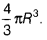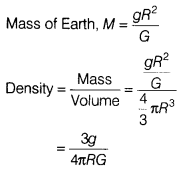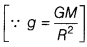• Let gravitational force be F when the distance between them is R,…(1)
Now, when the distance reduces to half,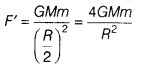…(2)
On dividing Eq. (1) by Eq. (2), we get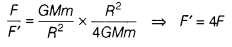• When the mass becomes 4 times,